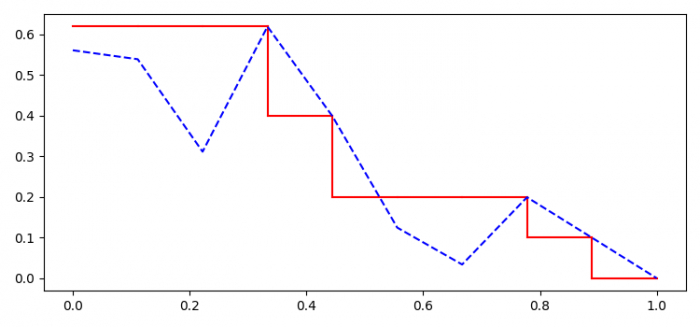# How to draw a precision-recall curve with interpolation in Python Matplotlib?

To draw a precision-recall curve with interpolation in Python, we can take the following steps −

## Steps

• Set the figure size and adjust the padding between and around the subplots.

• Create r, p and duplicate recall, i data points using numpy.

• Create a figure and a set of subplots.

• Plot the recall matrix in the range of r.shape.

• Plot the r and dup_r data points using plot() method.

• To display the figure, use show() method.

## Example

import numpy as np
import matplotlib.pyplot as plt

plt.rcParams["figure.figsize"] = [7.50, 3.50]
plt.rcParams["figure.autolayout"] = True

r = np.linspace(0.0, 1.0, num=10)
p = np.random.rand(10) * (1. - r)
dup_p = p.copy()
i = r.shape - 2

while i >= 0:
if p[i + 1] > p[i]:
p[i] = p[i + 1]
i = i - 1

fig, ax = plt.subplots()

for i in range(r.shape - 1):
ax.plot((r[i], r[i]), (p[i], p[i + 1]), 'k-', label='', color='red')
ax.plot((r[i], r[i + 1]), (p[i + 1], p[i + 1]), 'k-', label='', color='red')

ax.plot(r, dup_p, 'k--', color='blue')
plt.show()

## Output

It will produce the following output −